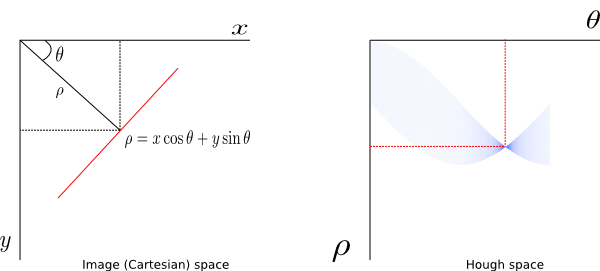NS

Linear Hough Transform Using Python

In this post I will explain the Hough transform for line detection. I will demonstrate the ideas in Python/SciPy.

Hough transform is widely used as a feature extraction tool in many image processing problems. The transform can be used to extract more complex geometric shapes like circles and ellipses but this post focuses on extracting lines in an image.

Quick Conceptual Review

In Cartesian coordinates, a line can be represented in slope-intercept form as$y=mx+c.$

The left panel in Fig. 1 shows a line (red color) in Cartesian coordinate system. The y-axis is chosen to be positive downward so that it matches with the commonly used image coordinate convention (top-left corner of an image is its origin). The value of$\theta$ is assumed positive when measured in clockwise direction from x-axis (to make the results comparable to MATLAB).Fig. 1: Representation of a line in Cartesian (left) and Hough (right) coordinates.

The equation of the line can be modified to get its alternate representation as$\rho=x\cos\theta+y\sin\theta$,

where$\rho$ is the distance of the line from the origin and and$\theta$ is the angle of the vector, of least magnitude,  to the line. The transformed coordinate space which has$\theta$ and$\rho$ as its axes, as shown in right panel of the above figure, is called Hough space. A point in image space is mapped to a sinusoidal curve in Hough space. A set of points belonging to a line in image space get mapped to a set of sinusoids intersecting at a point in Hough space. So the problem of detecting a line in an image becomes a problem of detecting a point in Hough space. Continue reading